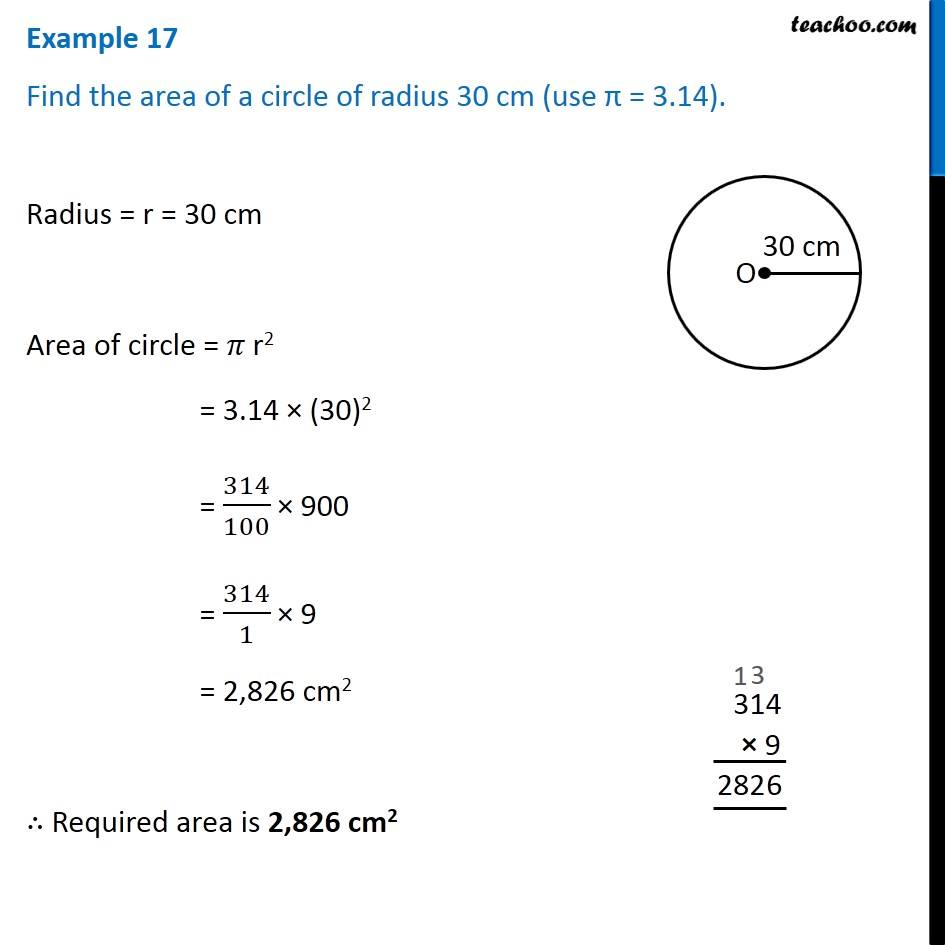Area of circle

Chapter 9 Class 7 Perimeter and Area
Concept wiseGet live Maths 1-on-1 Classs - Class 6 to 12

### Transcript

Example 12 Find the area of a circle of radius 30 cm (use π = 3.14). Radius = r = 30 cm Area of circle = 𝜋 r2 = 3.14 × (30)2 = 314/100 × 900 = 314/1 × 9 = 2,826 cm2 ∴ Required area is 2,826 cm2